## PHP 中函数 isset(), empty(), is_null() 的区别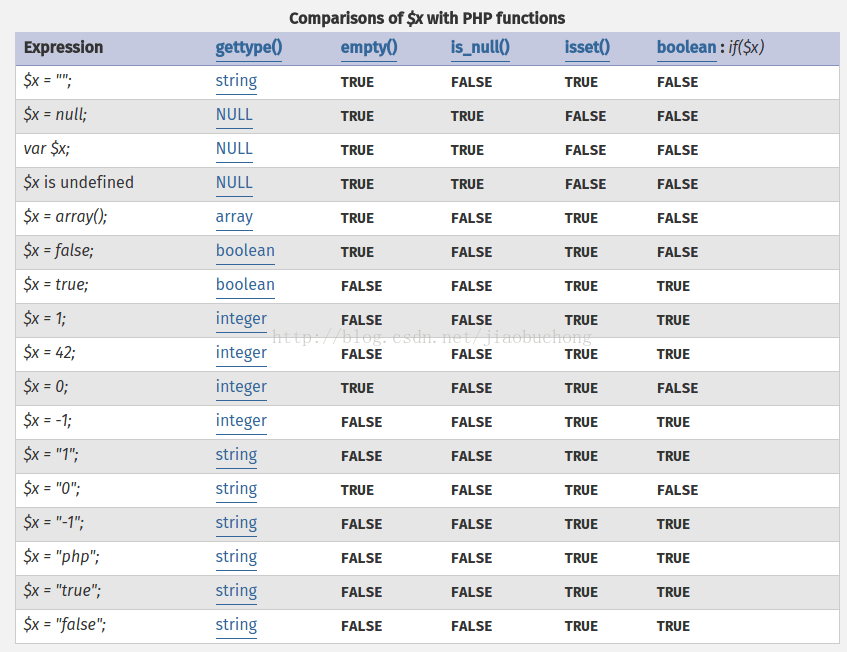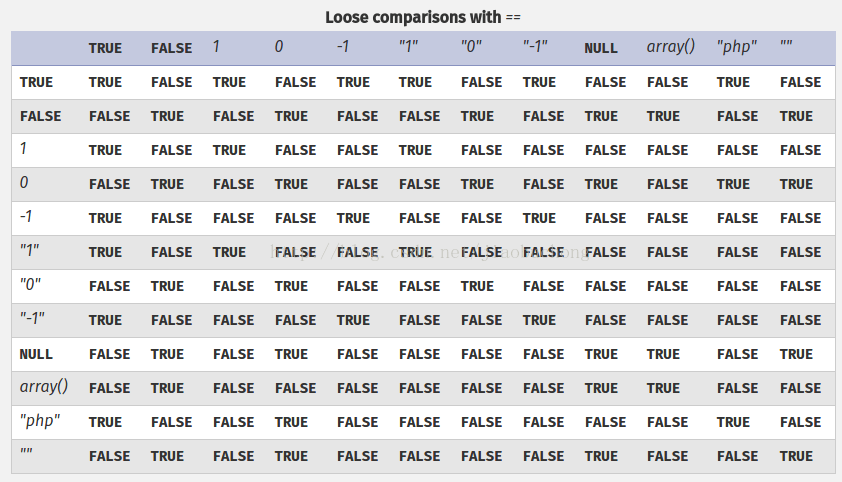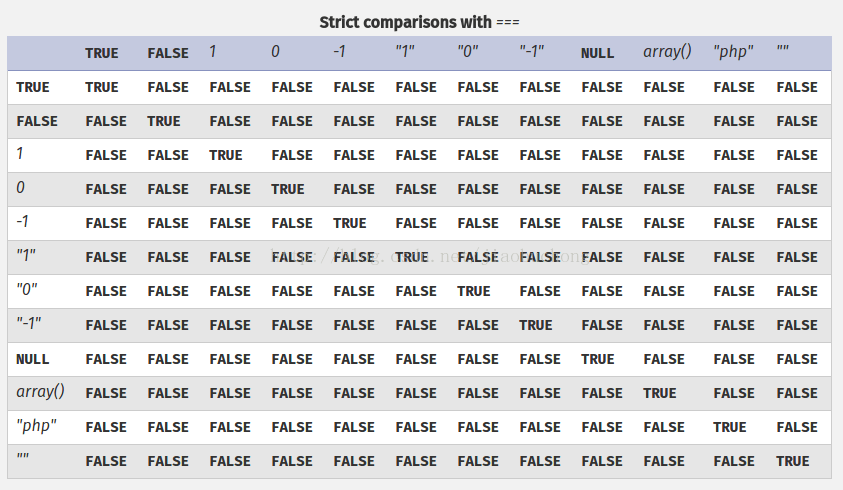### NULL:

```\$myvariable; //此处你想定义一个变量，但未赋值。会有Notice: Undefined variable
echo \$myvariable + 3; //使用这个变量出现：Notice: Undefined variable: myvariable in```

```\$myvariable = NULL;
echo \$myvariable + 3; //这样就不会有问题了```

### is_null()：

bool is_null ( mixed \$var ) (php.net官方文档的函数定义)

1、它被赋值为NULL
2、它还没有赋值
3、它未定义，相当于unset(),将一个变量unset()后，不就是没有定义吗

```\$myvar = NULL;
var_dump(is_null(\$myvar)); // TRUE
\$myvar1;
var_dump(is_null(\$myvar1)); // TRUE Notice: Undefined variable
\$num = 520;
unset(\$num);
var_dump(is_null(\$num)); //TRUE Notice: Undefined variable
var_dump(is_null(\$some_undefined_var)); //TRUE Notice: Undefined variable

\$myvar = 0; is_null(\$myvar); // FALSE
\$myvar = FALSE; is_null(\$myvar); // FALSE
\$myvar = ''; is_null(\$myvar); // FALSE```

### isset():

bool isset ( mixed \$var [, mixed \$… ] )，参数是一个变量

```\$myvar = NULL; isset(\$myvar); // FALSE 当一个变量被赋值为NULL时，就表示这个变量没有值
\$myvar = 0; isset(\$myvar); // TRUE
\$myvar = FALSE; isset(\$myvar); // TRUE
\$myvar = ''; isset(\$myvar); // TRUE
isset(\$some_undefined_var); // FALSE Undefined variable```

### defined()：

bool defined ( string \$name )

### empty():

bool empty ( mixed \$var )

```\$myvar = NULL; empty(\$myvar); // TRUE
\$myvar = 0; empty(\$myvar); // TRUE
\$myvar = FALSE; empty(\$myvar); // TRUE
\$myvar = ''; empty(\$myvar); // TRUE
empty(\$some_undefined_var); // TRUE```

empty()为TRUE的情况，若变量不存在，或者变量存在且其值为””、0、”0″、NULL、FALSE、array()、var \$var; 以及没有任何属性的对象，则返回 TURE。

## 写一个方法类，用于遍历某个文件夹下所有文件并显示

```<?php
function recursive(\$dir) {
\$handle = opendir(\$dir);
echo "<ul>";
if(\$line == '.' || \$line == '..') continue;
echo  "<li>".iconv('gbk','utf-8',\$line)."</li>";
if(is_dir("\$dir/\$line")){
recursive("\$dir/\$line");
}
}
echo "</ul>";
closedir(\$handle);
}
recursive("./")
?>
```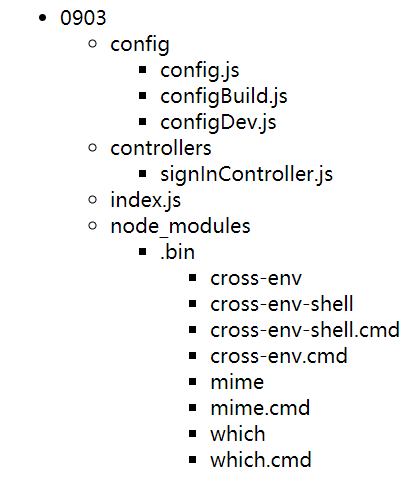## 用 JS 实现过滤 script 的正则表达式

```function stripscript(s) {
returns.replace(/<script.*?>.*?<\/script>/ig, '');
}```

/ 之间的内容 / 是 regex 正则语句的书写开始与结束

.*? 是贪婪的匹配 .* 是匹配任何字符，但用贪婪的就是不包含 > 的内容
/ig 是不区分大小写和全局替换

## PHP 数组查找函数 in_array()、array_search()、array_key_exists() 使用实例

### in_array(value,array,type)

```<?php
\$people = array("Peter", "Joe", "Glenn", "Cleveland");
if(in_array("Glenn", \$people)) {
echo"Match found";
}else{
}
?>```

`Match found`

### array_key_exists(key,array)

```<?php
\$a = array("a"=>"Dog", "b"=>"Cat");
if(array_key_exists("a", \$a)) {
echo"Key exists!";
}else{
echo"Key does not exist!";
}
?>```

`Key exists!`

### array_search(value,array,strict)

array_search() 函数与 in_array() 一样，在数组中查找一个键值。如果找到了该值，则返回匹配该元素所对应的键名。如果没找到，则返回 false。注意在 PHP 4.2.0 之前，函数在失败时返回 null 而不是 false。同样如果第三个参数 strict 被指定为 true，则只有在数据类型和值都一致时才返回相应元素的键名。
```<?php
\$a = array("a" => "Dog", "b" => "Cat", "c" => 5, "d" => "5");
echo array_search("Dog", \$a);
echo array_search("5", \$a);
?>```

`ac`

## PHP 数组 array_push() 和 array_pop() 以及 array_shift() 函数

```<?php
/**
* array_push() 将一个或多个单元压入数组的末尾(入栈)
*/
\$stack = array("Java", "Php", "C++");
array_push(\$stack, "C#", "Ruby", array('jsp', 'Asp'));
print_r(\$stack);
echo "====================================="."<br>";

/**
* array_pop() 将数组最后一个单元弹出（出栈）
*/
\$stack = array("Java", "Php", "C++", "C#", "Ruby");
array_pop(\$stack);
print_r(\$stack);
echo "====================================="."<br>";

/**
* array_shift() 将数组开头的单元移出数组 array_unshift() 在数组开头插入一个或多个单元
*/
\$stack = array("Java", "Php", "C++", "C#", "Ruby");
array_shift(\$stack);
print_r(\$stack);
?>```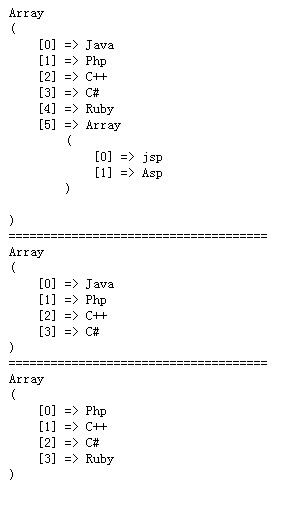## PHP 快速排序算法

```<?php
\$arr = array(6,5,32,8,4,5,1);
function quick_sort(\$arr){
if(!is_array(\$arr))return false;
\$length = count(\$arr);
if(\$length<=1)return \$arr;
\$left = \$right = array();
for(\$i = 1; \$i<\$length;\$i++){
if(\$arr[\$i]<\$arr){
\$left[] = \$arr[\$i];
}else{
\$right[] = \$arr[\$i];
}
}
\$left = quick_sort(\$left);
\$right = quick_sort(\$right);
return array_merge(\$left,array(\$arr),\$right);
}
echo "<pre>";
print_r(quick_sort(\$arr));
```

```Array
(
 => 1
 => 4
 => 5
 => 5
 => 6
 => 8
 => 32
)```

## 送给於总的视频-中建三局大连新星·星海中心## 中建三局大连新星星海中心项目中建总公司东北区域CI汇报## 大连新星·星海中心党群工作汇报5分38秒